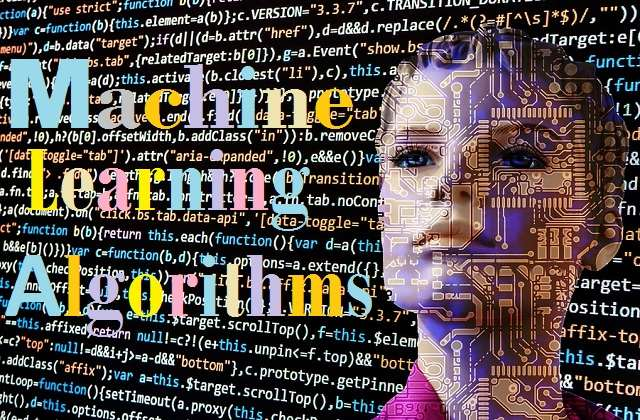## Friday, September 27, 2019Machine learning is an application of artificial intelligence (AI)

## What is Machine Learning and How Does Machine Learning Algorithm Work? | Applications of Machine Learning

### What is Machine Learning?

Machine learning is mainly a process in which machines like computers learn things on their own. In this way, computers acquire the ability to think and function like the human brain. In this process, they do not need any human help.
Basically, machine learning is a type of algorithm that helps software run correctly and is used to predict the outcomes without any programming. For this, it prepares a sample based on some of the results seen by the user and on the basis of that sample creates a pattern of future questions. A machine learning process is similar to data mining and predictive modeling processes. In both, the data is first searched in a pattern, and then it is applied according to the program.
Machine learning is also considered to be an application of artificial intelligence. Under this, through an idea, data is transmitted to a digital device in such a way that it can learn to work on its own.
The application of machine learning is continuously evolving over time. Many people are familiar with machine learning because they do online shopping from the internet and they see advertisements related to shopping, which also run with the help of machine learning.

#### How Does Machine Learning Work?

To understand how machine-learning works, it is very important to understand machine learning algorithms and their types. Different types of machine learning algorithms require different types of experts. In general, machine learning algorithms are of two types:

1. Supervised machine learning algorithms
2. Unsupervised machine learning algorithms

##### Supervised Machine Learning Algorithm
To create a supervised algorithm, we need a data scientist and data analyst who has complete knowledge of machine learning techniques and is able to create programs to make the machine work properly. He also explains accuracy and feedback in a big way. The data scientist tells which variable and features we should use for the development. As soon as its training is finished, the algorithm automatically applies to the new data. The data expert mainly looks at which variables and features should be used to construct this algorithm. This algorithm is automatically applied to new data as soon as this construction is complete. Thus it can be said that the supervised algorithm is constructed under the supervision or monitoring of the data expert.

##### Unsupervised Machine Learning Algorithm
The unsupervised algorithm does not require any training for any outgoing data. This is because it uses the interactive approach that we call deep learning.This algorithm is also known as Neural Networks.
This technique is mainly used in complex processing such as image recognition, speech to text and natural language generation. These types of neural networks connect millions of trained data automatically and form relationships with variables.
As soon as this data is trained, the new data easily does all the work using the algorithm and it is also useful to interpret the new data. This algorithm is also feasible in the work of big data because it requires a lot of data to be built.

##### What are the most popular machine learning algorithms?
Here is the list of 8 most commonly used machine learning algorithms:

###### 1. Naive Bayes Classifier Algorithm:
Naive Bayes Classifier Algorithm is a type of classification algorithms in Machine Learning based on Bayes' Theorem, which holds the assumption of independence among predictors.

###### 2. Decision Tree Algorithm:
A decision tree algorithm is a type of supervised learning algorithm that uses a tree-like model of decisions and their possible outcomes. The general purpose of using the decision tree algorithm is to build a training model that can estimate the value of a class or target variable by learning the decision rules inferred from the training data.

###### 3. K Means Clustering Algorithm:
K-means clustering algorithm makes an effort to divide the given anonymous data set into a fixed number (k) of the cluster. Initially, it identifies k number of centroids (the data point at the center of a cluster) and then allocates each data point to the nearest cluster.

###### 4. Support Vector Machine Algorithm:
A support vector machine (SVM) is a supervised learning model with associated learning algorithms that performs classification by detecting hyperplanes that maximize the difference between two classes.

###### 5. Linear Regression Algorithm:
The linear regression algorithm is a machine learning algorithm that performs regression tasks. This algorithm can be used to make predictions about a target variable based on the specific values of another variable. It is mostly used to detect the relationship between variables and forecasts.

###### 6. Logistic Regression Algorithm:
Logistic regression algorithm is a type of predictive analytics tools and techniques extracted from the field of statistics by machine learning. Logistic regression in its original form uses a logical operation to describe data and build up a binary dependent variable, then it is used to explain the relationship between a binary dependent variable and one or more nominal, interval, ordinal, or ratio-level independent variables.

###### 7. K-Nearest Neighbors Algorithm:
K-Nearest Neighbors is a non-parametric technique used for classification and regression in machine learning. It stores all available cases and classifies new ones based on the similarity scale.

###### 8. Artificial Neural Networks:
Artificial neural networks are computing systems based on the structures and functions of biological neural networks. In this technique, using training data set to construct the algorithm, a correlation is established between them. Thus the data is organized in such a way that it can subsequently divide the incoming data into groups and show all the data well.